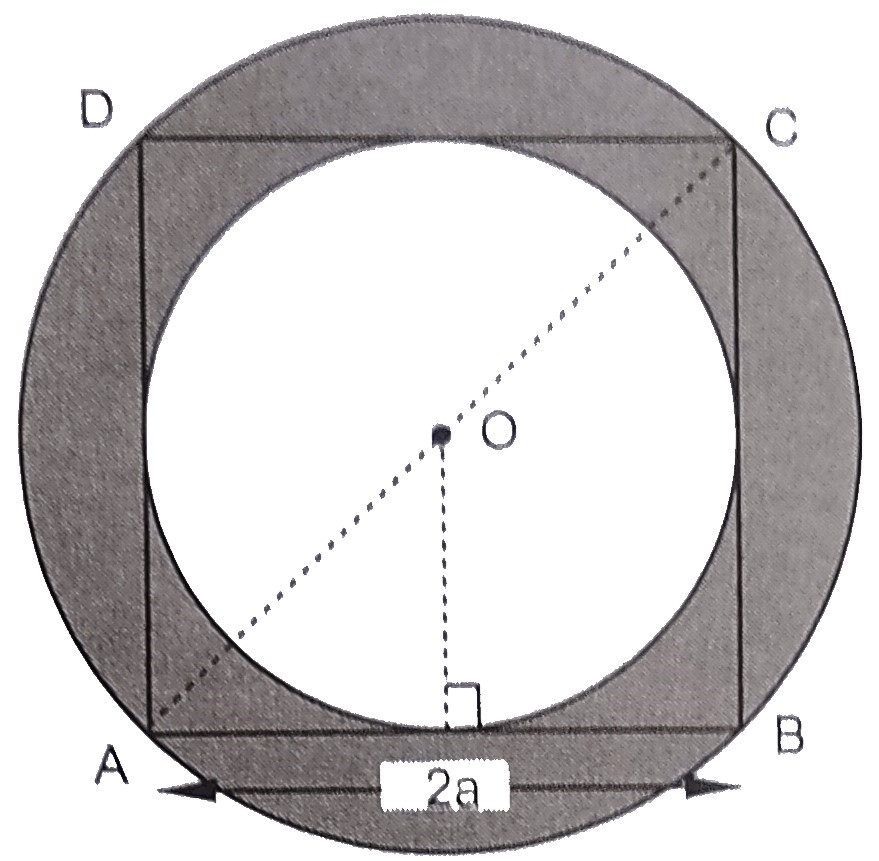"
">

# In the below figure, $A B C D$ is a square of side $2 a$. Find the ratio between the circumferences."

Given:

$A B C D$ is a square of side $2 a$.

To do:

We have to find the ratio between the circumferences.

Solution:

The square $ABCD$ is inscribed a circle.
Length of the side of the square $= 2a$

From the figure,

Diameter of the outer circle $AC =$ Diagonal of the square

$=\sqrt{2} \times 2 a$

$=2 \sqrt{2} a$

This implies,

Radius of the outer circle $R=\frac{\mathrm{AC}}{2}$

$=\frac{2 \sqrt{2} a}{2}$

$=\sqrt{2} a$

Diameter of the inner circle $=2a$

Radius of the inner circle $r=\frac{2a}{2}=a$

Therefore,

The ratio between the circumferences of the circles $=\frac{\text { circumference of outer circle }}{\text { circumference of inner circle }}$

$=\frac{2 \pi \mathrm{R}}{2 \pi r}$

$=\frac{\mathrm{R}}{r}$

$=\frac{\sqrt{2} a}{a}$

$=\frac{\sqrt{2}}{1}$

The ratio between the circumferences is $\sqrt{2}:1$.

Updated on: 10-Oct-2022

103 Views